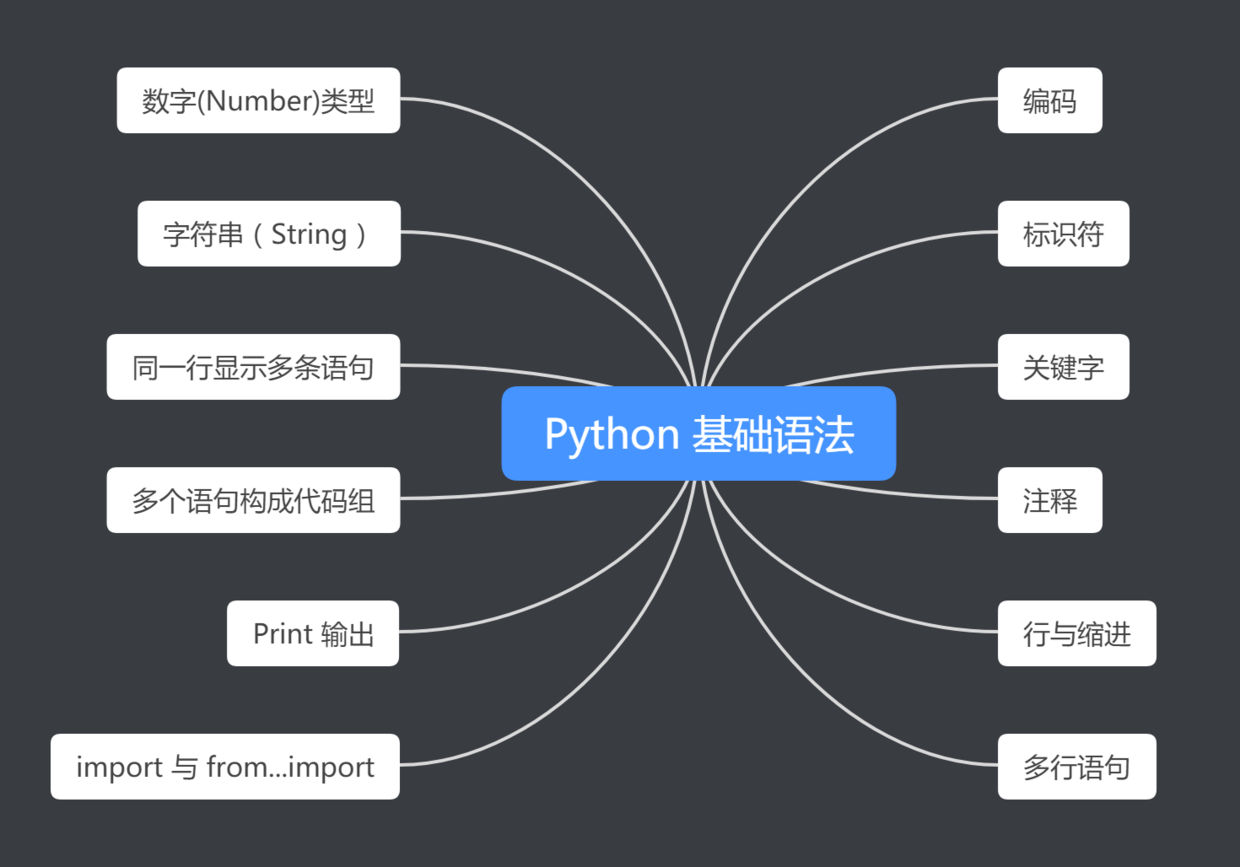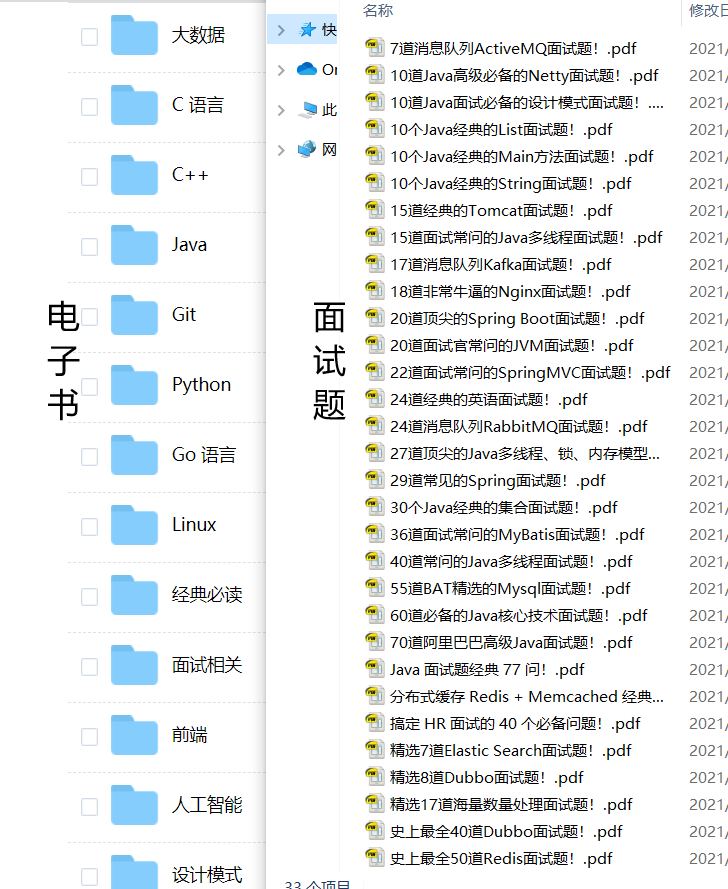## Python 实战（二）| 基础语法

|     |   0 浏览# 02 编码

``````# -*- coding: cp-1252 -*-
``````

# 03 标识符

Python 3 的标识符与 Java 中的一样：

# 04 关键字

Python 3 中的 keyword 模块，存储了 Python 3 中所有的关键字：

``````import keyword
print(keyword.kwlist)
['False', 'None', 'True', 'and', 'as', 'assert', 'break', 'class', 'continue', 'def', 'del', 'elif', 'else', 'except', 'finally', 'for', 'from', 'global', 'if', 'import', 'in', 'is', 'lambda', 'nonlocal', 'not', 'or', 'pass', 'raise', 'return', 'try', 'while', 'with', 'yield']
``````

# 05 注释

Python 3 与 Java 的注释大同小异。
Python 3 中的单行注释以 # 开头 如：

``````print("Hello world!") # 输出语句，输出 Hello world!
``````

Python 3 中的单行注释以 """ 开头，"""结尾 如：

``````"""

"""
print("Hello world!")
``````

####5、行与缩进
Python 使用缩进来表示代码块，不使用大括号，缩进的空格数可变（一般缩进 4 个空格），但必须包含相同的缩进空格数。正确格式如：

``````if True:
print ("True")
else:
print("False")

if True:
print ("True")
else:
print ("False")    # 缩进不一致，会导致运行错误
``````

``````IndentationError: unindent does not match any outer indentation level
``````

# 06 多行语句

Python 通常是一行写完一条语句，但如果语句很长，我们可以使用反斜杠()来实现多行语句，例如：

``````sum = x + \
y + \
z

sum = ['x','y','z',
'a','b']
``````

# 07 数字(Number)类型

Python 3 中数字有四种类型：整数、布尔型、浮点数和复数。
int (整数), 如 10, 只有一种整数类型 int，表示为长整型，没有 python2 中的 Long。

• bool (布尔), 如 True。
• float (浮点数), 如 1.23、3E-2
• complex (复数), 如 1 + 2j、 1.1 + 2.2j

# 08 字符串（String）

Python 3 中的单双引号使用完全相同

Python 中的字符串有两种索引方式，从左往右以 0 开始，从右往左以 -1 开始。
Python 没有单独的字符类型，一个字符就是长度为 1 的字符串。

``````str='Nasus'

print(str)                 # 输出字符串
print(str[0:-1])           # 输出第一个到倒数第二个的所有字符
print(str)              # 输出字符串第一个字符
print(str[2:4])            # 输出从第三个开始到第五个的字符
print(str[2:])             # 输出从第三个开始的后的所有字符
print(str * 2)             # 输出字符串两次
print(str + '你好')        # 连接字符串

print('------------------------------')

print('hello\nnasus')      # 使用反斜杠(\)+n转义特殊字符
print(r'hello\nnasus')     # 在字符串前面添加一个 r，表示原始字符串，不会发生转义
``````

``````Nasus
Nasu
N
sus
sus
NasusNasus
Nasus你好
---------------------------
hello
nasus
hello\nnasus
``````

# 09 同一行显示多条语句

Python 可以在同一行中使用多条语句，语句之间使用分号(;)分割，以下是一个简单的实例：

``````import sys; x = 'nasus'; sys.stdout.write(x + '\n')
``````

``````nasus
``````

# 10 多个语句构成代码组

``````if expression :
suite
elif expression :
suite
else :
suite
``````

# 11 Print 输出

print 默认输出是换行的，如果要实现不换行需要在变量末尾加上 end=""：

``````x="Hello"
y="world"
# 换行输出
print( x )
print( y )

print('---------')
# 不换行输出
print( x, end=" " )
print( y, end=" " )
print()
``````

``````Hello
world
---------
Hello world
``````

# 12 import 与 from...import

• 将整个模块(somemodule)导入，格式为： import somemodule
• 从某个模块中导入某个函数,格式为： from somemodule import somefunction
• 从某个模块中导入多个函数,格式为： from somemodule import firstfunc, secondfunc, thirdfunc
• 将某个模块中的全部函数导入，格式为：
``````from somemodule import *

# 导入 sys 模块
import sys
print('================Python import mode==========================');
print ('命令行参数为:')
for i in sys.argv:
print (i)
print ('\n python 路径为',sys.path)

# 导入 sys 模块的 argv,path 成员
from sys import argv,path  #  导入特定的成员

print('================python from import===================================')
print('path:',path) # 因为已经导入 path 成员，所以此处引用时不需要加 sys.path
``````

####后语# 13 大厂面试题 & 电子书